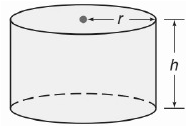Chapter 9.CT, Problem 12CT### Elementary Geometry for College St...

6th Edition
Daniel C. Alexander + 1 other
ISBN: 9781285195698

#### Solutions

Chapter
Section### Elementary Geometry for College St...

6th Edition
Daniel C. Alexander + 1 other
ISBN: 9781285195698
Textbook Problem
1 views

# For the right circular cylinder shown, r = 4 cm and h = 6 cm. Find the exacta) lateral area. __b) volume. _____

To determine

a)

To find:

The exact lateral area of the right circular cylinder.

Explanation

Given:

For the right circular cylinder r=4 cm, h=6 cm

Formula used:

The lateral area of the right circular cylinder is L=2πrh.

Calculation:

We have r=4,h=6

Substitute the known values in L=2πrh

To determine

a)

To find:

The exact volume of the right circular cylinder.

### Still sussing out bartleby?

Check out a sample textbook solution.

See a sample solution

#### The Solution to Your Study Problems

Bartleby provides explanations to thousands of textbook problems written by our experts, many with advanced degrees!

Get Started

#### Differentiate. f(z) = (1 ez)(z + ez)

Single Variable Calculus: Early Transcendentals, Volume I

#### True or False: If converges and converges, then converges.

Study Guide for Stewart's Single Variable Calculus: Early Transcendentals, 8th

#### The area of the region bounded by , , and r = sec θ is:

Study Guide for Stewart's Multivariable Calculus, 8th